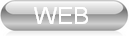﻿ Center of Modern Control Techniques and Industrial Informatics - Simulation Systems

Simulation Systems (SS)

 Course supervisor: doc. Ing. Anna Jadlovská, PhD. Lecturer: doc. Ing. Anna Jadlovská, PhD. Instructor: doc. Ing. Anna Jadlovská, PhD. Laboratory: PC FEI Study program: Cybernetics Degree: 1st (Bachelor) Grade: 2nd Semester: Winter

Course aim

The aim of this course is to familiarize students with the implementation possibilities for a variety of mathematical and physical problems using the Matlab/Simulink simulation language. The problems include the solution of linear and nonlinear algebraic equations, numerical solution of differential equations, data approximation by polynomials, data interpolation and 2D / 3D graphics, as well as modeling, identification and simulation of dynamical systems. The students are expected to become skilled in Matlab/Simulink, which is widely used in scientific and engineering calculations and data visualization. The goal of the course lies in the students mastering the numerical, graphical and modeling techniques integrated in the Matlab/Simulink software package and in the demonstration of the possibilities of using Control, Identification and Symbolic Toolboxes in teaching and in practical use.